# Empirical FormulaPage 1

#### WATCH ALL SLIDES

Slide 1Empirical Formula

From percentage to formula

Slide 2## The Empirical Formula

The lowest whole number ratio of elements in a compound.

The molecular formula the actual ratio of elements in a compound

The two can be the same.

CH2 empirical formula

C2H4 molecular formula

C3H6 molecular formula

H2O both

Slide 3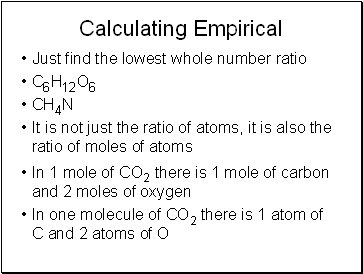## Calculating Empirical

Just find the lowest whole number ratio

C6H12O6

CH4N

It is not just the ratio of atoms, it is also the ratio of moles of atoms

In 1 mole of CO2 there is 1 mole of carbon and 2 moles of oxygen

In one molecule of CO2 there is 1 atom of C and 2 atoms of O

Slide 4Calculating Empirical

Pretend that you have a 100 gram sample of the compound.

That is, change the % to grams.

Convert the grams to mols for each element.

Write the number of mols as a subscript in a chemical formula.

Divide each number by the least number.

Multiply the result to get rid of any fractions.

Slide 5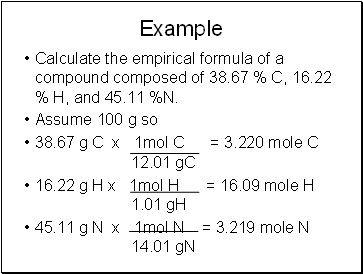Example

Calculate the empirical formula of a compound composed of 38.67 % C, 16.22 % H, and 45.11 %N.

Assume 100 g so

38.67 g C x 1mol C = 3.220 mole C 12.01 gC

16.22 g H x 1mol H = 16.09 mole H 1.01 gH

45.11 g N x 1mol N = 3.219 mole N 14.01 gN

Slide 63.220 mole C

16.09 mole H

3.219 mole N

C3.22H16.09N3.219

If we divide all of these by the smallest

one It will give us the empirical formula

Slide 7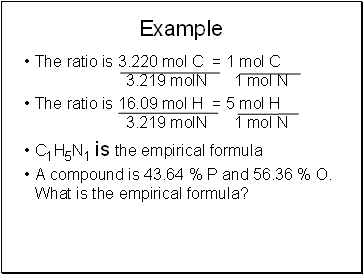Example

The ratio is 3.220 mol C = 1 mol C 3.219 molN 1 mol N

The ratio is 16.09 mol H = 5 mol H 3.219 molN 1 mol N

C1H5N1 is the empirical formula

A compound is 43.64 % P and 56.36 % O. What is the empirical formula?

Slide 8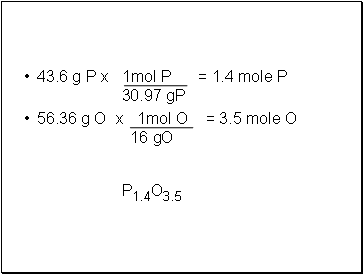43.6 g P x 1mol P = 1.4 mole P 30.97 gP

56.36 g O x 1mol O = 3.5 mole O 16 gO

P1.4O3.5

Slide 9## Divide both by the lowest one

The ratio is 3.52 mol O = 2.5 mol O 1.42 mol P 1 mol P

P1.4O3.5

P1O2.5

Slide 10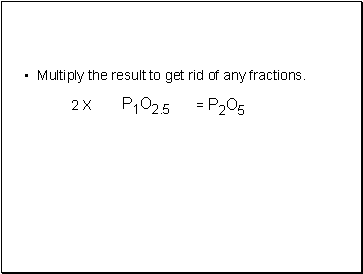Multiply the result to get rid of any fractions.

P1O2.5

2 X

= P2O5

Slide 11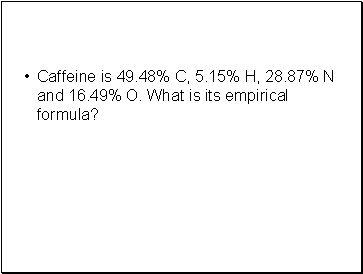Go to page:
1  2